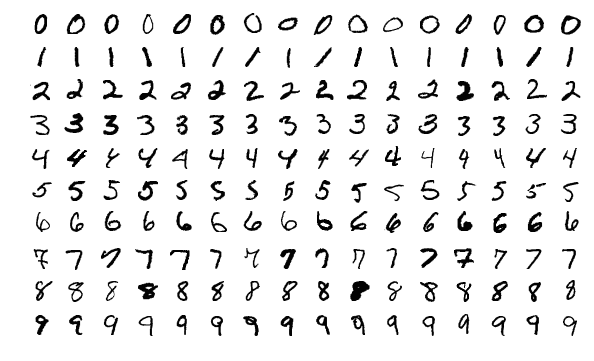## 3.4 MNIST Dataset

The MNIST dataset is a popular dataset for image classification machine learning model tutorials. It is conveniently included in the Keras library and ready to be loaded with build-in functions for analysis. The WIKI page of MNIST provides a detailed description of the dataset: https://en.wikipedia.org/wiki/MNIST_database. It contains 70,000 images of handwritten digits from American Census Bureau employees and American high school students. There are 60,000 training images and 10,000 testing images. Each image has a resolution of 28x28, and the numerical pixel values are in greyscale. Each image is represented by a 28x28 matrix with each element of the matrix an integer between 0 and 255. The label of each image is the intended digit of the handwritten image between 0 and 9. We cover the detailed steps to explore the MNIST dataset in the R and Python notebooks. A sample of the dataset is illustrated in the figure below:Sample of MNIST dataset (https://en.wikipedia.org/wiki/File:MnistExamples.png)